# Mixed math facts worksheets

## Mixed practice - math facts

These math facts worksheets provide mixed practice in the basic addition, subtraction, multiplication and division facts. Good practice for students who are close to mastering their math facts.

We also have mixed 4 operations worksheets with multi-digit numbers and thousands of math worksheets by grade level.

## Mixed addition and subtraction facts

Add & subtract within 10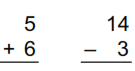Add & subtract - all facts
Add & subtract within 10 (horizontal)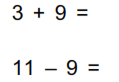Add & subtract - all facts (horizontal)

## Mixed multiplication and division facts

Multiply & divide smaller numbers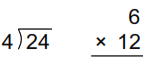Mixed multiplication and division facts
Multiply & divide (small numbers, horizontal)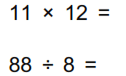Mixed multiplication and division facts (horizontal)
Missing factors and divisors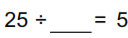## Mixed math facts (4 operations)

Mixed math facts (1-10)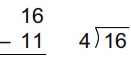Mixed math facts
Mixed math facts (horizontal, 1-10)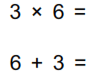Mixed math facts, horizontal

## Fact families

Fact families: add and subtract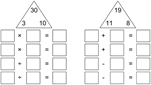Fact families: multiply and divide
Fact families: 4 operations

## Input / output charts

Input / output charts: add & subtract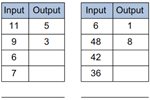Input / output charts: multiply & divide
Input / output charts: 4 operations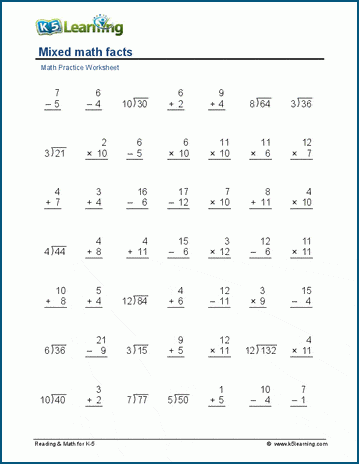Sample mixed math facts worksheet

What is K5?

K5 Learning offers free worksheets, flashcards and inexpensive workbooks for kids in kindergarten to grade 5. Become a member to access additional content and skip ads.